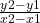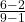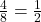## ??????? Anyone know? 7. What is the slope of the line that passes through the pair of points? (1, 2), and (9.6) (1 point) 0-1

Question

??????? Anyone know?
7. What is the slope of the line that passes through the pair of points? (1, 2), and (9.6) (1 point)
0-1
> 2

in progress 0
3 weeks 2021-08-31T14:20:29+00:00 1 Answers 0 views 0

slope =Step-by-step explanation:

Slope formula:Given points:

(1, 2) = (x1, y1)

(9, 6) = (x2, y2)

To find the slope of a line, input the values of two points into the slope formula:Solve:

6 – 2 = 4

9 – 1 = 8

Simplify:The slope is.